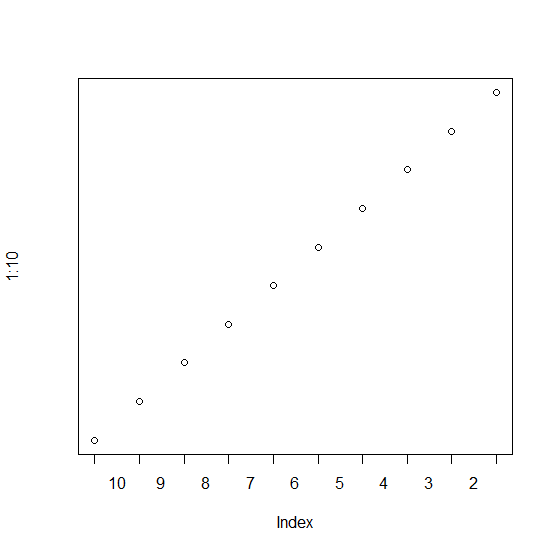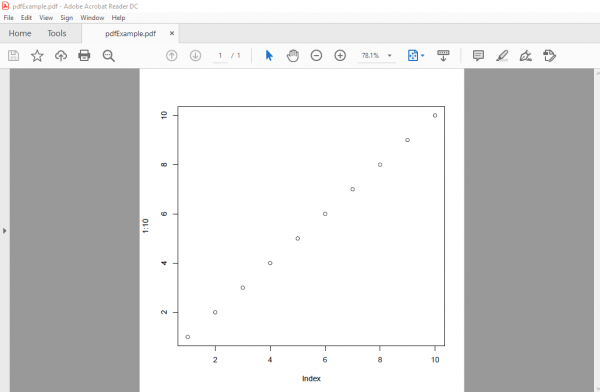# How to save a plot in pdf in R?

To save a plot in pdf, we can use the pdf function in base R. For example, if we want to save a plot with the name PDF then it can be done using the below command −

pdf("PDF.pdf")

After this we can create the plot and use dev.off().

## Example

pdf("pdfExample.pdf")
plot(1:10)

## Output## Example

Live Demo

dev.off()

## OutputTo check where it is saved, find the working directory using getwd().